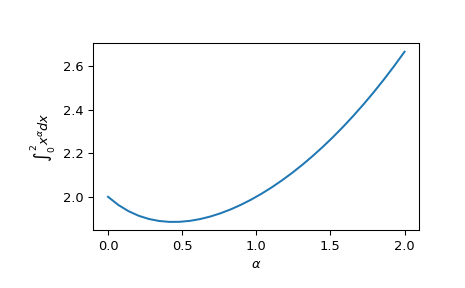# scipy.integrate.quad_vec¶

scipy.integrate.quad_vec(f, a, b, epsabs=1e-200, epsrel=1e-08, norm='2', cache_size=100000000.0, limit=10000, workers=1, points=None, quadrature=None, full_output=False)[source]

Adaptive integration of a vector-valued function.

Parameters
fcallable

Vector-valued function f(x) to integrate.

afloat

Initial point.

bfloat

Final point.

epsabsfloat, optional

Absolute tolerance.

epsrelfloat, optional

Relative tolerance.

norm{‘max’, ‘2’}, optional

Vector norm to use for error estimation.

cache_sizeint, optional

Number of bytes to use for memoization.

workersint or map-like callable, optional

If workers is an integer, part of the computation is done in parallel subdivided to this many tasks (using multiprocessing.pool.Pool). Supply -1 to use all cores available to the Process. Alternatively, supply a map-like callable, such as multiprocessing.pool.Pool.map for evaluating the population in parallel. This evaluation is carried out as workers(func, iterable).

pointslist, optional

List of additional breakpoints.

quadrature{‘gk21’, ‘gk15’, ‘trapz’}, optional

Quadrature rule to use on subintervals. Options: ‘gk21’ (Gauss-Kronrod 21-point rule), ‘gk15’ (Gauss-Kronrod 15-point rule), ‘trapz’ (composite trapezoid rule). Default: ‘gk21’ for finite intervals and ‘gk15’ for (semi-)infinite

full_outputbool, optional

Return an additional info dictionary.

Returns
res{float, array-like}

Estimate for the result

errfloat

Error estimate for the result in the given norm

infodict

Returned only when full_output=True. Info dictionary. Is an object with the attributes:

successbool

Whether integration reached target precision.

statusint

Indicator for convergence, success (0), failure (1), and failure due to rounding error (2).

nevalint

Number of function evaluations.

intervalsndarray, shape (num_intervals, 2)

Start and end points of subdivision intervals.

integralsndarray, shape (num_intervals, …)

Integral for each interval. Note that at most cache_size values are recorded, and the array may contains nan for missing items.

errorsndarray, shape (num_intervals,)

Estimated integration error for each interval.

Notes

The algorithm mainly follows the implementation of QUADPACK’s DQAG* algorithms, implementing global error control and adaptive subdivision.

The algorithm here has some differences to the QUADPACK approach:

Instead of subdividing one interval at a time, the algorithm subdivides N intervals with largest errors at once. This enables (partial) parallelization of the integration.

The logic of subdividing “next largest” intervals first is then not implemented, and we rely on the above extension to avoid concentrating on “small” intervals only.

The Wynn epsilon table extrapolation is not used (QUADPACK uses it for infinite intervals). This is because the algorithm here is supposed to work on vector-valued functions, in an user-specified norm, and the extension of the epsilon algorithm to this case does not appear to be widely agreed. For max-norm, using elementwise Wynn epsilon could be possible, but we do not do this here with the hope that the epsilon extrapolation is mainly useful in special cases.

References

 R. Piessens, E. de Doncker, QUADPACK (1983).

Examples

We can compute integrations of a vector-valued function:

>>> from scipy.integrate import quad_vec
>>> import matplotlib.pyplot as plt
>>> alpha = np.linspace(0.0, 2.0, num=30)
>>> f = lambda x: x**alpha
>>> x0, x1 = 0, 2
>>> y, err = quad_vec(f, x0, x1)
>>> plt.plot(alpha, y)
>>> plt.xlabel(r"$\alpha$")
>>> plt.ylabel(r"$\int_{0}^{2} x^\alpha dx$")
>>> plt.show()#### Previous topic

scipy.integrate.quad

#### Next topic

scipy.integrate.dblquad